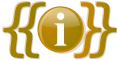# Template:Infobox shipDocumentation
```{{infobox ship
| name =
| image =
| description =
| weightcapacity =
| crewcapacity =
| speed =
| mastnumber =
| decknumber =
| type =
| category =
| health =
| fuel =
| weight =
| length =
| width =
| height =
| stacksize =
| itemid =
| blueprintpath =
| gfi =
| skilltree =
| engrampoints =
| craftingxp =
| craftingtime =
| prerequisite1 =
| prerequisite2 =
| prerequisite3 =
| unlocks =
| craftedin =
| craftedin2 =
| craftedin3 =
| craftingNr =
| quantity1 =
| ingredient1 =
| quantity2 =
| ingredient2 =
| quantity3 =
| ingredient3 =
| quantity4 =
| ingredient4 =
| quantity5 =
| ingredient5 =
| quantity6 =
| ingredient6 =
| quantity7 =
| ingredient7 =
| quantity8 =
| ingredient8 =
| quantity9 =
| ingredient9 =
| quantity10 =
| ingredient10 =
| quantity11 =
| ingredient11 =
| quantity12 =
| ingredient12 =
}}```